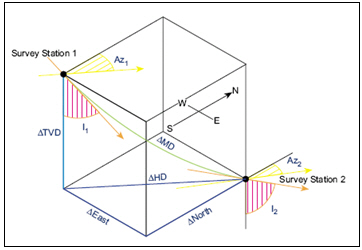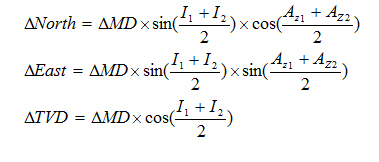# Angle Averaging Method in Directional Drilling Calculation

You can calculate North, East and TVD between directional surveys based on the Angle Averaging Method as per formula below:Where;

MD = measured depth between surveys in ft

I1 = inclination (angle) at upper survey in degrees

I2 = inclination (angle) at lower in degrees

Az1= Azimuth direction at upper survey

Az2 = Azimuth direction at lower survey

Calculation example for Angle Averaging Method

Survey 1

Depth = 7500 ft

Inclination = 45 degree

Azimuth = 130degree

Survey 2

Depth = 7595 ft

Inclination = 52 degree

Azimuth = 139 degreeAs per formula above,

North = -49.87 ft

East = 50.74 ft

TVD = 62.95 ft

Please find the Excel sheet used for directional survey calculation with Angle Averaging Method

Share the joy